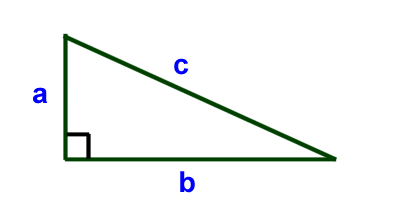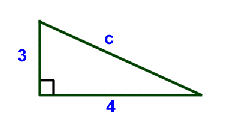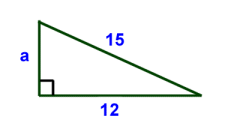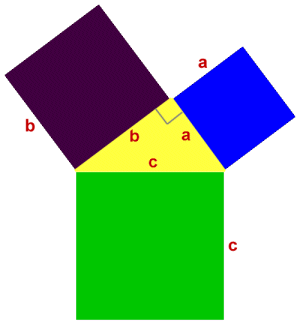# Kids Math

## Pythagorean TheoremSkills needed:
• Multiplication
• Exponents
• Square root
• Algebra
• Angles
The Pythagorean Theorem helps us to figure out the length of the sides of a right triangle. If a triangle has a right angle (also called a 90 degree angle) then the following formula holds true:

a2 + b2 = c2Where a, b, and c are the lengths of the sides of the triangle (see the picture) and c is the side opposite the right angle. In this example, c is also called the hypotenuse.

Let's work through a few examples:

1) Solve for c in the triangle below:

In this example a = 3 and b=4. Let's plug those into the Pythagorean Formula.

 a2 + b2 = c2 32 + 42 = c2 3x3 + 4x4 = c2 9+16 = c2 25 = c x c c = 52) Solve for a in the triangle below:

In this example b=12 and c= 15

 a2 + b2 = c2 a2 + 122 = 152 a2 + 144 = 225 Subtract 144 from each side to get: 144 - 144 + a2 = 225 - 144 a2 = 225 - 144 a2 = 81 a = 9The Pythagorean Theorem itself

The theorem is named after a Greek mathematician named Pythagoras. He came up with the theory that helped to produce this formula. The formula is very useful in solving all sorts of problems.

Here is what the theorem says:

In any right triangle, the area of the square whose side is the hypotenuse (remember this is the side opposite the right angle) is equal to the sum of the areas of the squares whose sides are the two legs (the two sides that meet at a right angle).

This may not make a lot of sense when you first read it. Let's show more of what the formula does and what the words say in a picture.

If you take each side of the yellow triangle and use it to make a square (see the picture below), then you get the three squares shown below. The area of each square is length x width. So in this example the area of each square is a2, b2, and c2.What the theorem says is that the area of the purple square plus the area of the blue square will equal the area of the green square. That's the same as saying:

a2 + b2 = c2

More Geometry Subjects

Circle
Polygons
Triangles
Pythagorean Theorem
Perimeter
Slope
Surface Area
Volume of a Box or Cube
Volume and Surface Area of a Sphere
Volume and Surface Area of a Cylinder
Volume and Surface Area of a Cone
Angles glossary
Figures and Shapes glossary

Back to Kids Math

Back to Kids Study